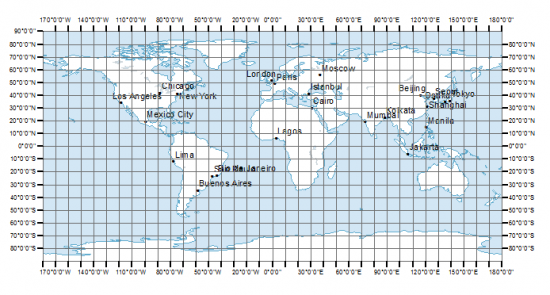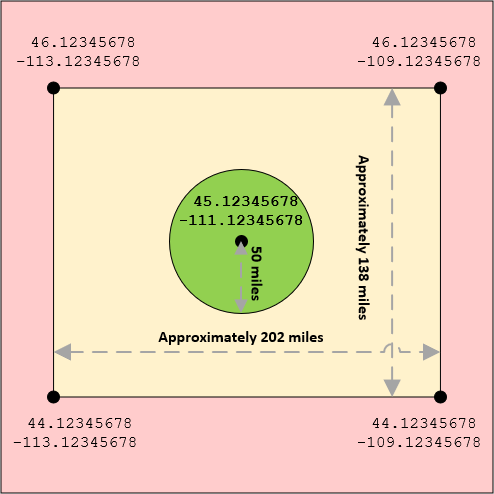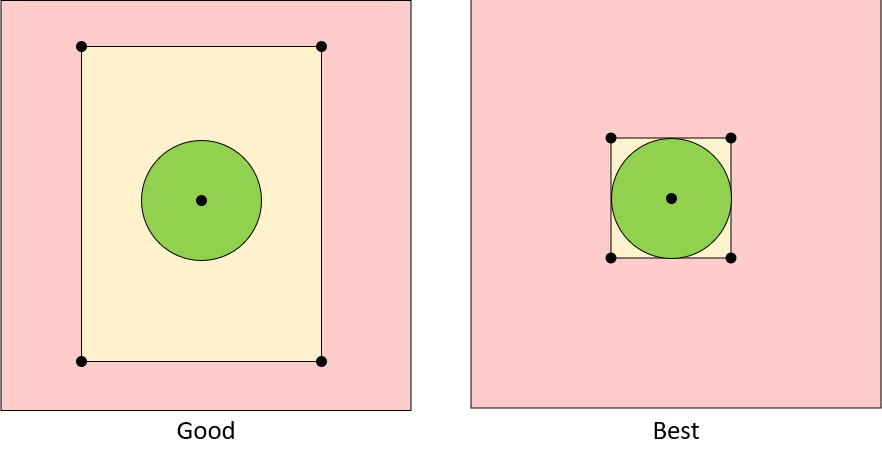# Locating Nearest Addresses with MySQL

Published:
Find out how to build "nearest location" capabilities using MySQL and GPS coordinates.
Whether it's locating the nearest store or finding the closest gas station, finding the nearest location within a certain distance is an extremely common request. Here, we'll talk about one of the most common ways to do it while retaining great performance and scalability.

Concepts in Distance Measurement
First thing you need is a very basic understanding of the concepts involved. First, we're all sitting on a sphere (sorry, flat-earthers, but it's true). Granted, it's not a -perfect- sphere, but it's pretty close, at least for the purposes of calculating distances between points. Those points are measured in latitude and longitude. These are measured in degrees but can be easily converted to decimals.

Here's what the measurements look like if you flattened out the earth (image courtesy of gisgeography.com):Latitude is represented by the horizontal lines (the lateral lines) and goes from -90 degrees (at the very bottom, so it's also called 90 degrees South) to 90 degrees (at the very top, so it's also called 90 degrees North).

Longitude is represented by the vertical lines and goes from -180 degrees (also referred to as 180 degrees West) to 180 degrees (180 degrees East).

If you look at the map above, you can see Los Angeles, CA is somewhere just around 120 degrees west, and around 35 degrees north. The way you might write this in degrees is 120°W and 35°N. If you were to go Google the exact GPS coordinates for the center of Los Angeles, you'd see that we're pretty close:

34° 3' 8.0460'' N and 118° 14' 37.2588'' W

The other numbers are minutes and seconds (34 degrees, 3 minutes, 8.0460 seconds). If you ever want to convert from degrees-minutes-seconds (DMS) to the decimal point, you:

1. Start with the degrees:
34

2. Divide the minutes by 60 (3 / 60 = 0.05) and add that:
34 + 0.05 = 34.05

3. Divide the seconds by 3600 (8.0460 / 3600 = 0.002235) and add that:
34.05 + 0.002235 = 34.052235

This might be helpful if you're dealing with a source of data that is represented in degrees, minutes, and seconds instead of decimals, BUT in our situation, it's better to use decimals, so when you're working with coordinates, always make sure you're storing and working with decimals.

Storing Latitude and Longitude in the Database
GPS coordinates can have a precision up to 8 possible decimal points, which means that if you are going to be storing latitude and longitude into the database, you'll probably want a DECIMAL field type and use DECIMAL(10,8) for latitude, and DECIMAL(11,8) for longitude (because longitude has one more possible digit). An example table schema:

```CREATE TABLE `locations` (
`location_id` INT UNSIGNED NOT NULL AUTO_INCREMENT,
`latitude` DECIMAL(10,8),
`longitude` DECIMAL(11,8),
PRIMARY KEY (`location_id`)
);```
For the purposes of this article, I used a script to put a million random entries into that locations table. If you want to follow along, here's a simple PHP script to do this fairly quickly:

```<?php
\$db = new mysqli("DB HOST HERE","USER NAME HERE","PASSWORD HERE","DATABASE NAME HERE");
\$db->autocommit(false);
\$stmt = \$db->prepare("INSERT INTO locations (latitude, longitude) VALUES (?, ?)");
\$stmt->bind_param('ss', \$latitude, \$longitude);
for(\$i = 0; \$i < 1000000; \$i++)
{
\$latitude = rand(-90,90) + (rand(0,59) / 60) + (rand(0,59) / 3600);
\$longitude = rand(-179,180) + (rand(0,59) / 60) + (rand(0,59) / 3600);
\$stmt->execute();
}
\$db->commit();```
Next thing is to find your own GPS coordinates (several sites offer this, one of them is https://www.maps.ie/coordinates.html). For demo purposes, I'm going to assume my latitude is 45.12345678 and my longitude is -111.12345678.

Finding Distance With Only the Database
The haversine formula can be performed within a SQL query, as shown below (I've underlined the parameters so you can see them a bit clearer):

```SELECT *, ROUND(
3959 * ACOS(
COS(RADIANS(45.12345678)) *
COS(RADIANS(latitude)) *
COS(RADIANS(longitude) - RADIANS(-111.12345678)) +
SIN(RADIANS(45.12345678)) * SIN(RADIANS(latitude))
)
,1) AS `miles_away`
FROM `locations`
HAVING `miles_away` <= 50
ORDER BY `miles_away````
The above will return all results within a 50-mile radius (the "3959" near the beginning of the query refers to the earth's radius of 6,371,000 meters, converted to miles)

Improving Performance
If I run this on my randomly-generated data set for my coordinates, I get 40 results and the query takes approximately 0.8 seconds. That might not seem like a long time, but I am running this on a database with no other traffic, minimal system I/O, a local database (eliminating all network transfer), and on a very fast SSD with lots of memory dedicated to the database and a powerful CPU, so if I were to run this in a far more average server scenario, it would likely take between 1 and 2 seconds or longer. So that's a pretty long query, especially if multiple people are executing it at the same time!

Why does it take so long? If we add an EXPLAIN to the beginning of the query and run it:

`EXPLAIN SELECT *, ROUND .... ORDER BY `miles_away``
You can see under the "rows" column that it looked at ALL 1,000,000 rows! In other words, it had to calculate the distance between my GPS coordinates and all 1,000,000 rows before it could figure out which ones were within a 50-mile radius.

So if we could reduce the number of records being evaluated, it would help performance by quite a bit! After all, do we really need to calculate the distance between the United States and Italy to know that it's not within 50 miles? If only 38 results are returned within 50 miles, then that means that 99.996% of the database is being filtered out.

So let's check out what kind of benefit we could get by indexing our latitude and longitude columns and putting a WHERE clause around them. First, we add the indexes:

```ALTER TABLE `locations`
ADD INDEX `longitude` (`longitude`),
ADD INDEX `latitude` (`latitude`);```
Now, every degree of latitude is just over 69 miles "tall" (so 45°N to 46°N is about 69 miles) and every degree of longitude is almost 49 miles (-111°W to -112°W is about 48.9 miles). So if we added a degree or two to each side of our current coordinates, we can create a very general "bounding box" can exclude MOST of the records in our locations table that are definitely WAY outside the 50-mile radius:

Current Latitude: 45.12345678
Current Longitude: -111.12345678

Here's the bounding box if we were to just add one degree above and below the latitude, and 2 degrees to the left and right of the longitude:So with our new indexes in place, let's see how fast the query is when we use our bounding box as additional criteria:

```SELECT *, ROUND(
3959 * ACOS(
COS(RADIANS(45.12345678)) *
COS(RADIANS(latitude)) *
COS(RADIANS(longitude) - RADIANS(-111.12345678)) +
SIN(RADIANS(45.12345678)) * SIN(RADIANS(latitude))
)
,1) AS `miles_away`
FROM `locations`
WHERE
`latitude` BETWEEN 44.12345678 AND 46.12345678
AND `longitude` BETWEEN -113.12345678 AND -109.12345678
HAVING `miles_away` <= 50
ORDER BY `miles_away````
With that simple change, suddenly the query starts coming back in 0.05 seconds instead of 0.8 seconds!

If we EXPLAIN that updated query, we can see that MySQL was able to use the longitude index to reduce the number of rows examined down to a fraction of the original number. On my randomly-generated data set, MySQL is now only examining 8,635 rows instead of all 1,000,000 rows. What an easy change that makes such a huge difference!

We Can Make It Even Faster!
What happens when 1,000 people are running this at once? What happens when the database has 10 million records or what if all of the records are densely packed within 100 miles where the bounding box isn't precise enough? We can make our bounding box even tighter to exclude even more undesired matches:So to do this, we use another series of formulas (if you're interested in the formulas themselves, you can find some excellent reference material here: http://www.movable-type.co.uk/scripts/latlong.html).

This time, we're looking for 4 points - 50 miles directly north of our current latitude, 50 miles directly east of our current longitude, 50 miles directly south of our current latitude, and 50 miles directly west of our current longitude.

In MySQL, the function for finding a new latitude, given a starting point is:

```DEGREES(ASIN(
SIN(RADIANS(<starting latitude>)) * COS(<miles>/3959) +
COS(RADIANS(<starting latitude>)) * SIN(<miles>/3959) * COS(RADIANS(<bearing>))
))```
The same function, except for longitude:

```DEGREES(RADIANS(<starting longitude>) + ATAN2(
SIN(RADIANS(<bearing>)) * SIN(<miles>/3959) * COS(RADIANS(<starting latitude>)),
COS(<miles>/3959) - SIN(RADIANS(<starting latitude>)) * SIN(RADIANS(<starting latitude>))
))```
The bearing is in degrees, like a clock, where 0 degrees is directly north, 45 degrees is northeast, 90 degrees is directly east, 180 degrees is directly south, and 270 degrees is directly west.

So if we want to find the latitude exactly 50 miles north of our current latitude of 45.12345678, the SQL would be:

```DEGREES(ASIN(
SIN(RADIANS(45.12345678)) * COS(50/3959) +
COS(RADIANS(45.12345678)) * SIN(50/3959) * COS(RADIANS(0))
))```
Longitude for 50 miles directly east of -111.12345678 (with the latitude of 45.12345678 and bearing of 90 degrees):

```DEGREES(RADIANS(-111.12345678) + ATAN2(
SIN(RADIANS(90)) * SIN(50/3959) * COS(RADIANS(45.12345678)),
COS(50/3959) - SIN(RADIANS(45.12345678)) * SIN(RADIANS(45.12345678))
))```
With these formulas, we can calculate the edges of our bounding box exactly 50 miles out from the center:

```SELECT *, ROUND(
3959 * ACOS(
COS(RADIANS(45.12345678)) *
COS(RADIANS(latitude)) *
COS(RADIANS(longitude) - RADIANS(-111.12345678)) +
SIN(RADIANS(45.12345678)) * SIN(RADIANS(latitude))
)
,1) AS `miles_away`
FROM `locations`
WHERE
`latitude` BETWEEN
DEGREES(ASIN(SIN(RADIANS(45.12345678)) * COS(50/3959) + COS(RADIANS(45.12345678)) * SIN(50/3959) * COS(RADIANS(180))))
AND
DEGREES(ASIN(SIN(RADIANS(45.12345678)) * COS(50/3959) + COS(RADIANS(45.12345678)) * SIN(50/3959) * COS(RADIANS(0))))
AND
`longitude` BETWEEN
DEGREES(RADIANS(-111.12345678) + ATAN2(SIN(RADIANS(270)) * SIN(50/3959) * COS(RADIANS(45.12345678)), COS(50/3959) - SIN(RADIANS(45.12345678)) * SIN(RADIANS(45.12345678))))
AND
DEGREES(RADIANS(-111.12345678) + ATAN2(SIN(RADIANS(90)) * SIN(50/3959) * COS(RADIANS(45.12345678)), COS(50/3959) - SIN(RADIANS(45.12345678)) * SIN(RADIANS(45.12345678))))
HAVING `miles_away` <= 50
ORDER BY `miles_away````
The result is an instant improvement from 0.5 seconds to about 0.25 seconds! If we EXPLAIN the latest query, we now see that the # of rows being examined/calculated has dropped from 8,635 down to 5,264!

The benefits of using indexes with the bounding box are enormous when it comes to scalability and performance.

Oh, and Use SQL_NO_CACHE...
One final thing we should do is add the SQL_NO_CACHE keyword to our SELECT query:

`SELECT SQL_NO_CACHE *, ROUND ....etc....`
Why? Because the query cache is used to hold commonly-executed queries. More often than not, you're using different starting coordinates every single time (different people checking different distances). There's very little point in filling up the query cache with query results that will probably not be seen or used again. It's better to leave that query cache memory available to other queries that truly deserve to be cached.

Plus, with our bounding box optimizations, the results should return so quickly anyway that we wouldn't get many benefits from the cache even if the exact same query were executed again (a visitor refreshing a page of results later on).

Yikes, that's... a LOT of SQL!
Yes, the above query is decently large and would be hard to comprehend if you were to revisit it the following year. So you may want to consider creating the above as a reusable stored procedure with parameters, like this:

```DELIMITER \$\$

CREATE PROCEDURE GetLocationsWithinXMiles(IN param_Latitude DECIMAL(10,8), IN param_Longitude DECIMAL(11,8), IN param_WithinXMiles DECIMAL(8,4))
BEGIN
SELECT SQL_NO_CACHE *, ROUND(
3959 * ACOS(
COS(RADIANS(param_Latitude)) *
COS(RADIANS(latitude)) *
COS(RADIANS(longitude) - RADIANS(param_Longitude)) +
SIN(RADIANS(param_Latitude)) * SIN(RADIANS(latitude))
)
,1) AS `miles_away`
FROM `locations`
WHERE
`latitude` BETWEEN
DEGREES(ASIN(SIN(RADIANS(param_Latitude)) * COS(param_WithinXMiles/3959) + COS(RADIANS(param_Latitude)) * SIN(param_WithinXMiles/3959) * COS(RADIANS(180))))
AND
DEGREES(ASIN(SIN(RADIANS(param_Latitude)) * COS(param_WithinXMiles/3959) + COS(RADIANS(param_Latitude)) * SIN(param_WithinXMiles/3959) * COS(RADIANS(0))))
AND
`longitude` BETWEEN
DEGREES(RADIANS(param_Longitude) + ATAN2(SIN(RADIANS(270)) * SIN(param_WithinXMiles/3959) * COS(RADIANS(param_Latitude)), COS(param_WithinXMiles/3959) - SIN(RADIANS(param_Latitude)) * SIN(RADIANS(param_Latitude))))
AND
DEGREES(RADIANS(param_Longitude) + ATAN2(SIN(RADIANS(90)) * SIN(param_WithinXMiles/3959) * COS(RADIANS(param_Latitude)), COS(param_WithinXMiles/3959) - SIN(RADIANS(param_Latitude)) * SIN(RADIANS(param_Latitude))))
HAVING `miles_away` <= param_WithinXMiles
ORDER BY `miles_away`;
END \$\$

DELIMITER ;```
Once the stored procedure is created, you can just execute it with a very easy-to-understand CALL query:

`CALL GetLocationsWithinXMiles(45.12345678, -111.12345678, 50);`
Couldn't I Do This Client-Side?
All of this geo-calculation stuff is all easily done on either the database OR the client-side (e.g. PHP code). In theory, you could definitely pull back all the records within the bounding box, and then calculate it with PHP instead:

```SELECT SQL_NO_CACHE *
FROM `locations`
WHERE
`latitude` BETWEEN DEGREES(...blah blah...) AND DEGREES(...blah blah...)
AND
`longitude` BETWEEN DEGREES(...blah blah...) AND DEGREES(...blah blah...)```
However, is that truly better? Probably not. People who know me know that I advocate for doing as much processing as possible on the client-side rather than using DB resources. However, in this case, let's think about what happens and do some tests.

If we do the calculations on the DB side, the query runs in about 0.25 seconds and about 40 ready-to-use results are transferred back to the client.

If we did the calculations on the client-side code, the DB would first have to transfer all the matches within the bounding box. In this case, there were a total of 56 possible matches in the bounding box, which means that it would be up to the client-side code to evaluate the 56 distances and exclude the 16 that were outside the 50-mile radius.

That means we're transferring a bit of data from the database that is essentially garbage and we're expecting the client to figure out which records are to be thrown away. That's a waste of bandwidth. It may not be very noticeable at such a low number of records like 56, nor when your database service and web service are running on the same physical server, but it can become more visible once you start separating the services onto separate servers and have longer distances to check.

And as far as the query performance goes, the uncached result still came back in about 0.25 seconds on average, so there was no large performance gain from getting the raw data instead of having the DB do the calculations.

If your database server is even remotely decently-powerful, then it will probably be more efficient to run the calculations on the database side. The only time this would probably be different is if your database server was severely under-powered and your web server was far more capable, but you'd have to test your specific scenario and compare.

Copyright © 2020 - Jonathan Hilgeman. All Rights Reserved.
1
284 Views

#### Comments (0)

Have a question about something in this article? You can receive help directly from the article author. Sign up for a free trial to get started.

Get access with a 7-day free trial.